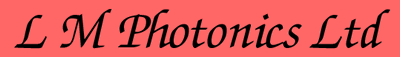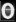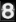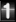how to calculate the shaft speed of induction motors, how to calculate the output power of an induction motor, formula for designing induction motor, Ac induction motor calculation question, induction motor calculations, ac motor calculations questions, how to calculate shaft speed in induction motors, Induction motor calculation, induction motor calculation, single phase induction motor design calculations, formula for kw of induction motor, shaft power of an inductor motor, Shaft power formula in an induction motor, Single phase motor calculations

Home  Books  Software  Power factor  Motor Control  Motor Starters  Soft Starters  Variable Speed  Advertise  Energy Savers

© L M Photonics Ltd | P.O. Box 13 076, Christchurch, New Zealand | phone : (NZ) +64 274 363 067